## Law of Sines and Cosines

Trig has been our trusty old friend whenever we have needed to find a missing side or angle in a right triangle, but where's the love for non-right triangles? Just because they don't have a right angle doesn't mean we can just brush them aside and forget that they have sides and angles and feelings too.

Well, that ends today. We're going to give them all the attention they deserve. Probably even more attention than they're comfortable with. It's going to be awkward for everyone involved, and we apologize in advance.

• ### Law of Sines

Triangles teach trig.

Right angles always around.

Old bonds break away.

Yep, we've starting things off with a haiku, and there's nothing you can do to stop us. Now we're going to use trig on non-right triangles, and finally lift the right-angle restriction we've been operating under since day 1.

So, let's look at a non-right triangle, shall we?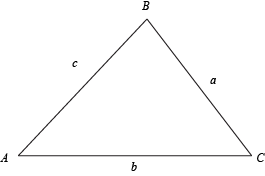What a-cute triangle. To understand non-right triangles and how we can use trig with them, we need to create a few right triangles within this guy. We'll be done comparing them to right triangles soon, we promise.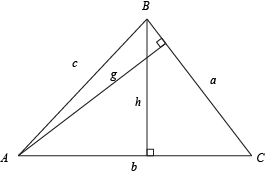Let's start investigating the height, h, using these right triangles. We did this a bit last time, when we found the new area formula for triangles.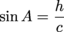h = c sin A

We're not stopping here, though. We can find h another way, using C.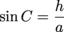h = a sin C

Two we set these two equal, because we can totally do that.

c sin A = a sin C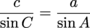We now have a trig equation relating sides and angles of a non-right triangle. But B is feeling left out. We can fix that, though, by using the height g just like we did h.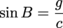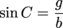Which, with some rearranging gives us: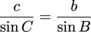See how our assistant,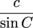, has shown up a second time? With its help, we're ready for the grand reveal, the Law of Sines: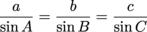Ta-da. This says that the proportions of each angle and its opposite side are equal throughout a triangle. We can now use trig to find missing sides or angles from all kinds of triangles, not just right triangles. Think of it as our eye in the sky searchlight when hunting for a missing piece.

### Sample Problem

A triangle has a 65° angle with the side opposite that angle measuring 18 units. What is the measurement of the side opposite a 32° angle in the same triangle?

The Law of Sines shows us the way. We only need two of the equalities, not all three.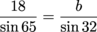We keep each side and angle paired together: a with A, and b with B. We can then rearrange things to solve for b: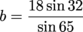But, uh, how are we going to deal with those angles? Double angles, half angles, none of them look any good. Well, we're breaking our unspoken rule and giving out some non-standard angles now that we're in the last section. Yep, dust off those calculators, make sure they're set for "Degree" mode, and get ready to calculate.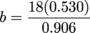= 10.52 units

Using the calculator again felt good. We missed you buddy.

Sometimes we'll be looking for an unknown angle instead of a side. We're lazy, and so to avoid an extra step, we can rewrite the Law of Sines by dividing the whole thing by 1.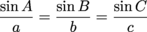This is the same formula, just flippy-flopped upside down. Just don't drop it while spinning it around.

• ### Law of Cosines

It's time for more triangle shenanigans. The Law of Sines works great when we can use it, but it has a pretty glaring flaw. Like Achilles and his heel, the Law of Sines is vulnerable in its weak point: we have to know at least one of the triangle's angles. No angle, no luck.

That's where the Law of Cosines comes in. Let's look at another triangle to see where it comes from.

The law, not the triangle. Guess we should have been clearer there. We don't really know where the triangle comes from. We just pulled it from our mysterious drawer full of triangles.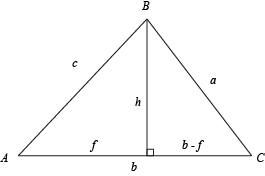When deriving the Law of Sines, we came up with: h = c sin A. In the same way, we can say that f = c cos A, since f is the side adjacent to angle A.

Here's where things take a turn for the weird. Let's focus on the smaller triangle on the right (the one with the sides a, h, and b- f). Why? We're not really sure, we just know it all works out in the end. We tried asking a mathematician, but he just threw down a smoke bomb and ran off in the confusion.

The thing is, we want to know about the sides of the triangle, and we already have an equation for that for the smaller triangle: the Pythagorean Theorem.

h2 + (b - f)2 = a2

We know another way of writing h and f that includes sine and cosine.

(c sin A)2 + (bc cos A)2 = a2

It's time to work some magic with algebra, starting with the squares. Warning: hairy-looking equation ahead. Get out your trimmers and wade into the thick of it:

c2 sin2 A + (b2 – 2bc cos A + c2 cos2 A) = a2

c2 sin2 A + c2 cos2 A + b2 – 2bc cos A = a2

c2 (sin2 A + cos2 A) + b2 – 2bc cos A = a2

Almost done, then we can try and get rid of all this hair. For the next step, we need the Pythagorean Identity: sin2A + cos2A = 1.

c2(1) + b2 – 2bc cos A = a2

Now, after much wading, we have the Law of Cosines:

a2 = b2 + c2 – 2bc cos A

This works for all three angles/sides in a triangle, so we can also say:

b2 = a2 + c2 – 2ac cos B

c2 = a2 + b2 – 2ab cos C

We've got 4 variables in these equations: the three sides of the triangle and any one of the angles. As long as we know three of these things, we can suss out the fourth. Yes, we'll suss it.

It'd be a good idea to memorize at least one of these equations. They're kind of important. Here's something to help you out: look at whichever variable is alone. For instance, when it's a2, then the last term, -2bc cos A, doesn't have an a in the "-2bc" part, but it does have an angle A inside the cosine.

### Sample Problem

A triangle has sides measuring 4, 7, and 10 units. What is the measurement of the angle opposite the side measuring 7 units?

To start off, we need names for these sides and angles. We like Barkley, Shannon, Lee, and Archibald Williamson the Third, but we should probably pick some more math-y names. How about a = 4, b = 7, c = 10, and B for the angle opposite b? They're rather traditional, but they work.

The Law of Cosines gives the equation:

b2 = a2 + c2 – 2ac cos B

We can start plugging stuff into here and simplifying, so that we can find Archibald Williamson the Third. Er, B.

72 = 42 + 102 – 2(4)(10)cos B

49 = 16 + 100 – 80cos B

-67 = -80cos B

0.8375 = cos B

Now that cos (Archibald Williamson the Third)—sorry, cos B—is isolated, we can find the measurement of B by taking the inverse. Using our calculator in this case.

B = cos-1 (0.8375)

B ≈ 33.1°

Thank you, Law of Cosines, for helping us track down Archibald Williamson the Third. This really is a better name for the angle than B, don't you think? Now that we've found it, it just looks like an "Archibald Williamson the Third."

### Sample Problem

A triangle has sides measuring 13 units and 16 units, with an included angle of 42°. What is the length of the side opposite the 42° angle?

We can also use Law of Cosines to find a missing side opposite a known angle, as long as we know the other two sides. Check it:

a2 = b2 + c2 – 2bc cos A

Our mystery side is a, and we have A = 42, b = 13, and c = 16. Let's put those numbers in where they belong:

a2 = 132 + 162 – 2(13)(16)cos 42

All that is left to do here is chug on through, then take a square root to get the value of a:

a2 = 169 + 256 – 416(0.743)

a2 = 115.85

a = 10.8 units

And with that, the mystery is solved. Take that, Sherlock, we don't need you. How about you go back in the van with Shaggy and Scooby.